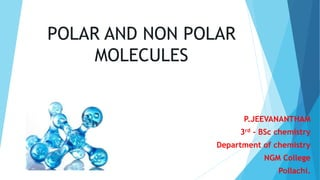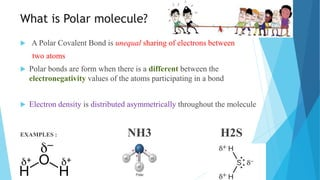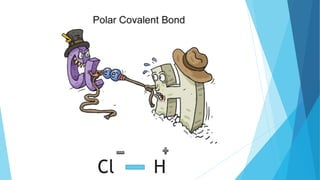Successfully reported this slideshow.

# Polar and non polar compounds and dipole moment - PPT×

# Polar and non polar compounds and dipole moment - PPT

This power point work describe about polar and nonn polar compounds and how to find it very easily and it also explain dipole moment and its calculation...this includes some workout problems

This power point work describe about polar and nonn polar compounds and how to find it very easily and it also explain dipole moment and its calculation...this includes some workout problems

### Polar and non polar compounds and dipole moment - PPT

1. 1. POLAR AND NON POLAR MOLECULES P.JEEVANANTHAM 3rd - BSc chemistry Department of chemistry NGM College Pollachi.
2. 2. What is Polar molecule?  A Polar Covalent Bond is unequal sharing of electrons between two atoms  Polar bonds are form when there is a different between the electronegativity values of the atoms participating in a bond  Electron density is distributed asymmetrically throughout the molecule EXAMPLES : NH3 H2S
3. 3. Cl H
4. 4. What is Non Polar molecule?  A Polar covalent is EQUAL sharing of electrons between two atoms  Polar bonds are form when there is a similar the electronegativity values of the atoms participating in a bond.  Electron density is distributed symmetrically within the molecule  EXAMPLE :  H2 Cl2 BF3 
5. 5. H H
6. 6. Classification of Bonds You can determine the type of bond between two atoms by calculating the difference in electronegativity values between the elements The bigger the electronegativity difference the more polar the bond.
7. 7. Practice What type of bond is HCl ? (H = 2.1, Cl = 3.1) Difference = 3.1 – 2.1 = 1.0 Therefore it is polar covalent bond. Your Turn To Practice  N(3.0) and H(2.1) = 0.9 (polar)  Al(1.5) and Cl(3.0) = 1.5 (polar)  Mg(1.2) and O(3.5) = 2.3 (ionic)  H(2.1) and F(4.0) = 1.9 (polar)  H(2.1) and H(2.1) = 0 (non polar)  Ca(1.0) and Cl(3.0) = 2 (ionic)
8. 8. Summary  In a polar bond, one atom is more electronegative than the other.  In a nonpolar bond, both atoms have similar electronegativity's.  An asymmetric molecule with polar bonds is a polar molecule.  An asymmetric molecule with nonpolar bonds is a nonpolar molecule.  A symmetric molecule, regardless of the polarity of the bonds, is always a nonpolar molecule.
9. 9. Polar = A,B,E Non polar = C,D Questn 1 Example: Identify each of the following compounds as either a polar molecule or a nonpolar molecule. A. NH3 B. CHCl3 C. CO2 D. BF3 E. CO
10. 10. DIPOLE MOMENT AND APPLICATION OF DIPOLE MOMENTS IN STUDY OF SIMPLE MOLECULE
11. 11. The product of magnitude of negative or positive charge(q) and the distance between the centres of the positive and negative charges is called dipole moment. It is usually donated by µ.  A dipole moment is a quantity that describes two opposite charges separated by a distance OR  If a molecule has a dipole moment,then we call it “polar”.  Dipole moment is a vector quantity i.e. it has magnitude as well as direction.
12. 12. DRAW DIPOLE MOMENT Dipole moment is a vector quantity and is represented by a small arrow with tail at the positive center and head pointing towards a negative center. For example, the dipole moment of HCl molecule is 1.03 D and the dipole of HCl may be represented as:
13. 13. FORMULA  A quantitative measures of the polarity of a bond its dipole moment. μ which is the product of the charge Q and the r is distance between the charges  Q is charge of electron = 1.60217662 × 10-19 coulombs r is a distance µ = Q × r 1 D = 3.335 × 10-30 C m (SI units)
14. 14. EXAMPLE Carbon tetrachloride m = 0 D Carbon tetrachloride has no dipole moment because all of the individual bond dipoles cancel.
15. 15. m = 1.62 D The individual bond dipoles do not cancel in dichloromethane; it has a dipole moment.
16. 16. Greater the electronegativity difference between the bonded atom, greater if the dipole moment. Dipole moment of hydrogen halides are in the order : H-F > H-Cl > H-Br > H-I
17. 17. APPLICATION 1)In determining the polarity of bonds : Greater is the magnitude of dipole moment,higher will be the polarity of the bond. This is applicable to molecules containing only one polar bond. In case of non-polar molecules like H2 , O2, N2 etc, the dipole moment is found to be zero . This is because there is no charge separation in these molecules. 2)In determining the symmetry of the molecules If any molecule possesses two or more polar bonds ,it will not be symmetrical if it possesses some molecular dipole moment. Water µ= 1.84 D H2S µ = 0.95 D NH3 µ=1.47 D
18. 18. If a molecule contains a number of similar atoms linked to the central atom and the overall dipole moment of the molecule is found to be zero ,this will imply that the molecule is symmetrical. For Ex: BF3 , CH4 , CCl4 4) To distinguish between cis and trans isomer Cis isomer usually has higher dipole moment than trans isomer 5)To distinguish between Ortho, meta and para isomers The dipole moment of para isomer is zero and that of ortho is greater than that of meta.
19. 19. SUMMARY  Molecular dipole depends on the geometry of the molecule  When the molecule does not possess a net dipole moment, the molecule is nonpolar.  When the molecule possesses a net dipole moment, the molecule is polar.